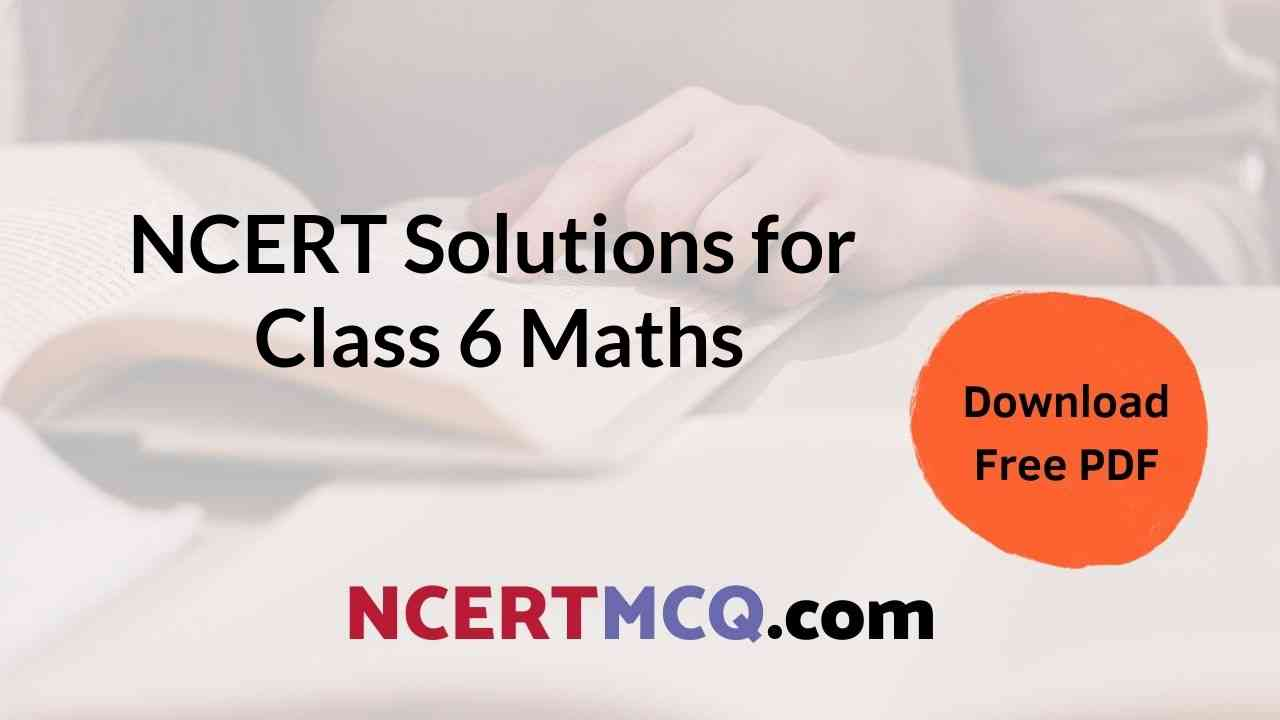From this page, students of CBSE class 6 can download chapter-wise NCERT Solutions for Class 6 Maths in pdf and know the answers to all the questions. It gives detailed explanations for all the questions presented in the NCERT 6th class maths textbooks guided by the Central Board of Secondary Education(CBSE). All solutions covered in the 6th Class Maths NCERT Solutions pdf make you understand the concepts easily and get a good grip on the subject. So, download the NCERT Solutions of 6th class mathematics pdf for better preparation.

## Free PDF Download of Chapter-wise NCERT Textbook Solutions of 6th Class Mathematics

NCERT CBSE Maths Solutions for Class 6 are given here for all 15 chapters in pdf form. Go through the pdf quick links provided below and download chapter-wise NCERT 6th Class Maths Solutions Pdf for free. Get them offline and start preparing all questions & answer well in the annual exams. All these solutions are written by our subject experts as per the CBSE syllabus and guidelines. So, download & aid your maths exam preparation.

### Advantages of Solving Class 6 Maths NCERT Solutions PDF

Here are some of the benefits of solving NCERT Solutions for class 6 maths pdf. Check out the advantages and kickstart your preparation effectively.

• Learninsta offered chapter-wise NCERT Class 6 Maths Solutions pdf aid students to crack the board exam by offering an in-depth understanding of the topics.
• Also, Students can clear their doubts using NCERT Textbook Solutions of 6th Class Maths.
• You can practice the different types of questions covered in the NCERT Maths Textbook by using CBSE Class 6 Maths NCERT Solutions Pdf.
• It builds a strong foundation of all these concepts for higher-level classes.
• Moreover, you can make use of this NCERT solution pdf for quick revision before the exam.

### FAQs on Maths NCERT Class 6 Solutions for All Chapters

1. What is the best study material for solving Chapter-wise 6th Class Maths Problems?

NCERT Solutions for Class 6 Maths is the best study material to understand the concepts and solve each and every problem easily and quickly.

2. Class 6 Students can refer to NCERT Textbook Solutions for exam preparation?

Yes, CBSE 6th Class students can refer to the NCERT textbook solutions for better exam preparation. You can use these solutions for preparing all subjects like Maths, Science, Social Science, English & Hindi.

3. Where can I find chapter-wise NCERT class 6 mathematics solutions for free?

Learninsta.com is a reliable and trusted website where you can easily & freely get all chapters of NCERT class 6 mathematics solutions in pdf format. All these study resources are accessible online and download offline.

### Final Words

Keep in touch with Learninsta.com and get the updated academic materials like NCERT Solutions for Class 6 Maths, and other subjects & prepare well for your final examinations. Also, you can find various study resources like Previous papers, books, quiz questions, etc. for all chapters of 6th Class Maths.